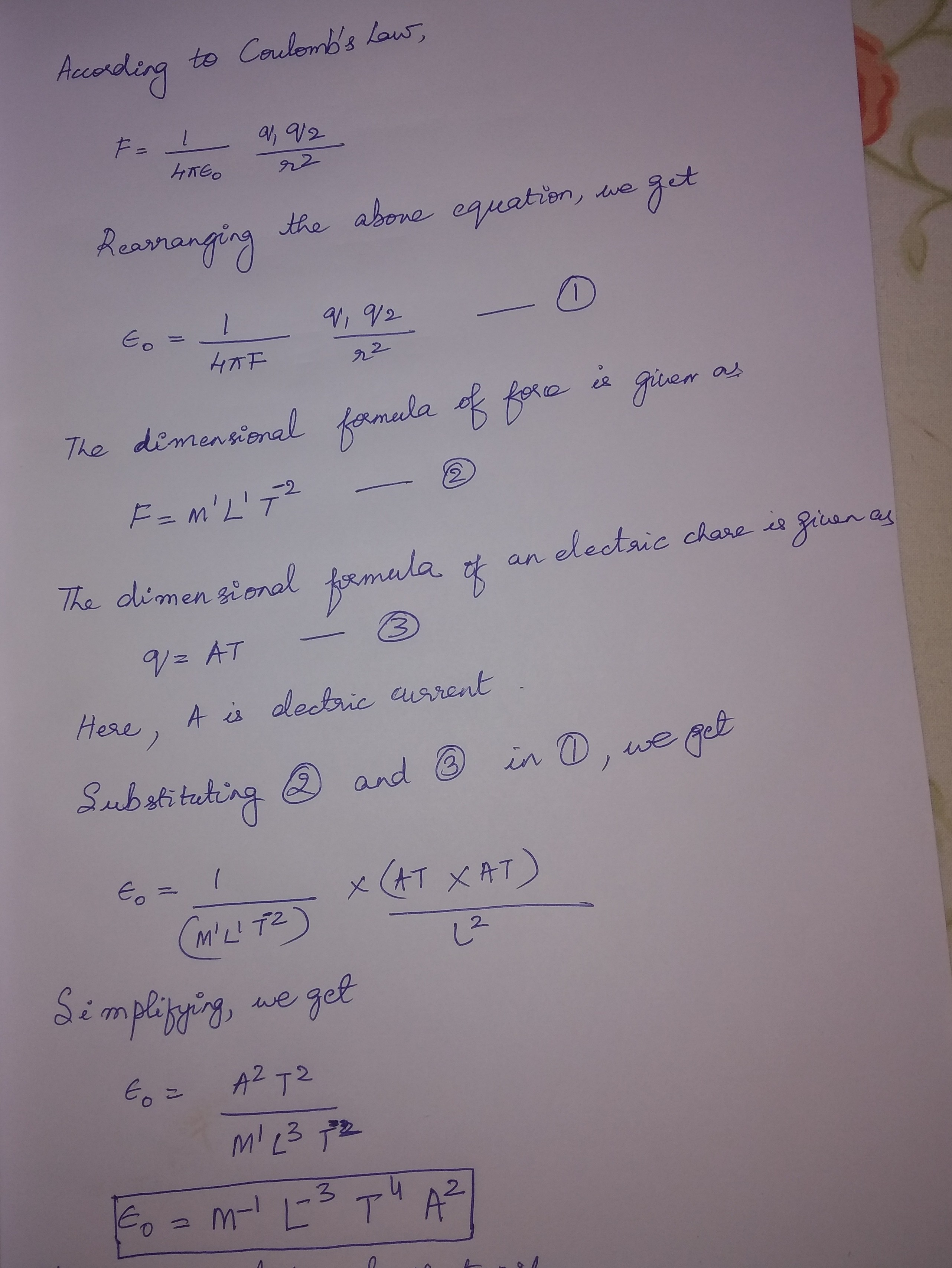Jet Set Go! All about Aeroplanes Jet Set Go! All about Aeroplanes

# Epsilon Naught Value

Epsilon Naught is synonymous to the permittivity of free space or absolute permittivity or electric constant, represented by the Greek alphabet ε0. The Epsilon Naught value is constant at any part of the universe. It is often miss-used as the value of Epsilon not. Permittivity is the measure of the opposition offered against the formation of an electric field.

## Value Of Epsilon Naught

The permittivity of free space(ε0) is the capability of the classical vacuum to permit the electric field. It as the definite defined value which can be approximated to

ε0 = 8.854187817 × 10-12 F.m-1 (In SI Unit)

Or

ε0 = 8.854187817 × 10-12 C2/N.m2 (In CGS units)

## Epsilon Naught Units

The permittivity of free space(ε0 ) can be expressed using the SI unit as well as CGS units. Following table brief the Epsilon unit –

 Epsilon Naught Units Units Epsilon Naught in SI Farad per meter or F.m-1 Epsilon Naught in CGS Columb square per Newton meter squared or C2/N.m2

### Epsilon Naught Physics

In physics, the Epsilon Naught value has good significance. Some of them are listed below.

• It represents the dielectric permittivity of the free space.
• The value of Epsilon Naught is used in calculating the dielectric constant of a material.

Hope you got to know the Epsilon naught along with the Epsilon naught unit in SI unit and CGS unit, its significance and its applications in various parts of physics.

Physics Related Topics:

Stay tuned with BYJU’S for more such interesting articles. Also, register to “BYJU’S-The Learning App” for loads of interactive, engaging physics-related videos and unlimited academic assistance.

## Frequently Asked Questions – FAQs

### What is epsilon naught?

Epsilon Naught is synonymous with the permittivity of free space or absolute permittivity or electric constant. It is represented by the Greek alphabet ε0.

### What is permittivity?

In electromagnetism, permittivity is the measurement of the electric polarisability of a dielectric.

### What is meant by the permittivity of space?

The permittivity of free space ε0 is the capability of the classical vacuum to permit the electric field. It is defined as the value which can be approximated to
$$\begin{array}{l}\varepsilon _{0} = 8.854187817 \times 10^{-12} F.m^{-1} \end{array}$$

### What are the units of Epsilon Naught?

In SI units, it is Farad per meter.
In CGS units, Coulomb squared per Newton meter squared.

### What is the significance of Epsilon Naught in Physics?

It represents the dielectric permittivity of the free space.
The value of Epsilon Naught is used in calculating the dielectric constant of a material.
Test your knowledge on Epsilon value